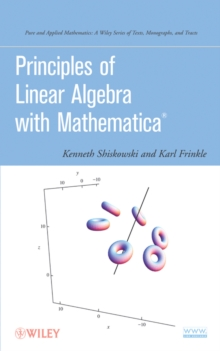Supporting your high street Find out how »
• My AccountPrinciples of Linear Algebra with Mathematica Hardback

Part of the Pure and Applied Mathematics: A Wiley Series of Texts, Monographs and Tracts series

Description

This hands-on introduction to the theoretical and computational aspects of linear algebra using Mathematica uniquely bridges the gap between beginning linear algebra and computational linear algebra that is often encountered in applied settings.

The commands required to solve complex and computationally challenging problems using Mathematica are provided, as well as coverage of linear systems of equations and matrices, applications of linear systems and matrices, determinants, inverses, and Cramer's rule.

This book is an ideal reference for students and professionals who would like to gain a further understanding of the use of Mathematica to solve linear algebra problems.

Information

• Format: Hardback
• Pages: 616 pages
• Publisher: John Wiley & Sons Inc
• Publication Date:
• Category: Mathematics
• ISBN: 9780470637951

£104.00

£92.69

on all orders

Pick up orders

from local bookshops

£105.00

£93.55